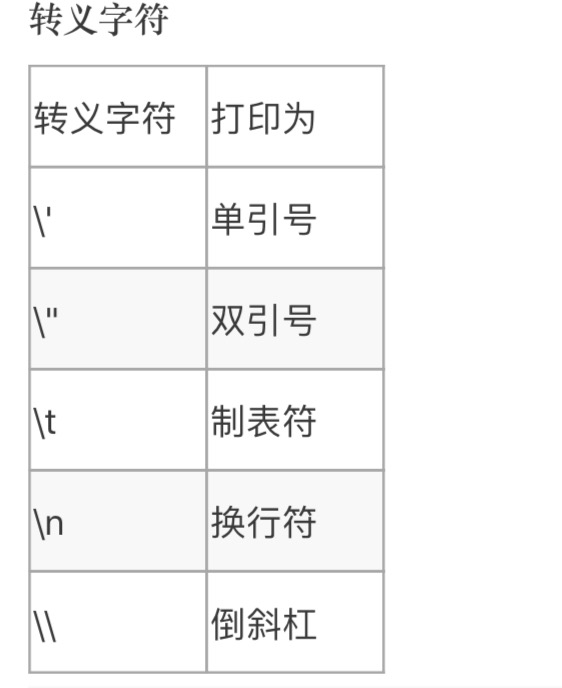《金哥百变Python零基础教程8》~字符串

# 本章知识点 :> `字符串`# 字符串

• 现在我们看看字符串的更多特性
• 本章相对来说说非常简单（只是一些知识的汇总)

• `123``”123“` 是两种类型的变量

• 在python里，有一个函数是可以查看变量类型的，叫`type(变量)`

• `t1 = type(123)`
`t2 = type("123")`
`print(t1)`
`print(t2)`

试一下 在线Python
• 因为Python里的运算对变量类型要求是严格的，所以有时候会因为类型不对报错

• 你可以用type函数看一下具体的类型是不是你预期的

# 转义字符

• 我们看一个特殊的字符串 `'That is Alice's cat.'`
• 它特殊在哪呢，我们注意到字符串里有一个 `'` 单引号
• 如果直接把 `'` 单引号 写在字符串里，python会报错

`s = 'That is Alice's cat.'`

• 为什么会这样呢？
• 我们要把它拆开来看 `'That is Alice'`      `s cat.'`
• 我故意把字符串拆开了
• 你会发现python很难识别到底你要写几个字符串，它只要按你要写2个来对待了，但是两个也不对啊
• 第二个字符串缺少 引号啊，于是报错了

• 怎么解决这个问题？

• 我们可以用 `\'` 转义 表示字符串里的 `'`

`s = That is Alice\'s cat.'`

• 需要转义表示的还有多个符号# 字符串与列表

• 现实中有一个问题是很常见的，字符串存储了一篇文章，而文章很长（有多行)
• 这个时候 `字符串` 的多行表示方法可以方便的定义一个多行字符串
• ``` ''' #3引号开头 Dear Alice, Eve's cat has been arrested for catnapping, cat burglary, and extortion. Sincerely, Bob ''' #3引号结尾 ```
• 你猜的没错，这个方法就叫 `'''` 3引号法
试一下 在线Python

• 还记得之前我们用for循环遍历字符串吗？

`s = '我是中国人'`
`for w in s:`
`print(w)`

`list = ['我', '是', '中', '国', '人']`
`for w in list:`
`print(w)`

• `s``list` 都可以这样遍历，其实字符串的内部结构就是这样的
• 换句话说，你把字符串看做单字符组成的列表是没有问题的
• 因此，你可以猜猜下面的操作会是什么结果？

`w = s #还记得列表的操作吗`
`print(w)`

• len函数

`l = len(s)`
`print(l)`

• 取中间的一段 (列表也有这样的操作，虽然没有讲)

`s1 = s[0:2]`
`print(s1)`

# 格式化

• 什么是格式化？
• 在字符串使用中经常有这样的场景，`'我今年' + str(20)`
• 或者 `'我的名字叫' + name`
• 简单的理解为，一个字符串它不是静态的，而是随时会变的
• 或者说：这个字符串是一个模板（类似excel模板)
• 具体数据在模板上填写
• 这样的情况可以用格式化的方法来简化。

• 具体语法

• `age = 20`
`name = '金哥'`
`s = '我今年 %d, 我的名字叫 %s' % (age, name)`
`print(s)`
`s = '我今年 %d, 我的名字叫 %s' % (age+1, name)`
`print(s)`
*中间的 `%` 表示要做格式化
• '我今年 %d, 我的名字叫 %s' 就是我们说的模板
• 第一个`%d` 和 第二个`%s`是占位符，`%d` 表示将来这里要填整数 `%s` 将来要填字符串
试一下 在线Python

# 字符串函数

• find 字符串查找
• `s = ’abcefg‘`
`i = s.find(b)`
`print(i)`
• `s.find(b)` 表示从`s`里查找`b`的位置。

• join() 和 split()

• `# 如果有几个字符串'aa', 'bb', 'cc'`
`# 可以用用join函数把他们连到一起`
`s = ' '.join(['aa' , 'bb', 'cc'])`
`print(s)`
`#s的结果 "aa bb cc" #注意，中间的空格就是join前面的空格`
`s = 'x'.join('aa' , 'bb', 'cc'])`
`print(s)`
`#s结果是axxbbxcc #即：用x来连接他们`

试一下 在线Python
• split()非常好懂，就是join()的反过程

• `s = 'aa bb cc'`
`l = s.split(' ')`
`print(l)`
`s = 'axxbbxcc'`
`l = s.split('x')`
`print(l)`

试一下 在线Python

# 综合运用• 我们看一下之前定义的地铁结构
• ```d1 = {'四惠东':'四惠','四惠':'大望路','大望路':'国贸','国贸':'永安里','永安里':'建国门', '建国门':'东单','东单':'王府井','王府井':'天安门东','天安门东':'天安门西','天安门西':'西单','西单':'复兴路', '复兴路':'南礼士路','南礼士路':'木樨地'}```
• 再看另一个结构

• `d1 = '四惠东,四惠,大望路,国贸,永安里,建国门,东单,王府井,天安门东,天安门西,西单,复兴路,南礼士路,木樨地'`
• 有没有发现，第二种结构显然跟接近我们描述一条线路，一个用逗号分隔开的字符串。

• 但是第二个虽然适合人类理解，但不适合我们的算法，怎么办？
• 问题：如果把第二种结构（字符串）转化为第一种结构(字典)

• 命名：`地铁线路转化器`

• 思路：字符串可以用 split方法先转成 列表
• 循环这个列表，把当前站和下一站作为 键值对
• `d1 = '四惠东,四惠,大望路,国贸,永安里,建国门,东单,王府井,天安门东,天安门西,西单,复兴路,南礼士路,木樨地'`
`def s2d(d1):`
`l = []`
`d = {}`
`l = d1.split(",")`
`n = 0 #这个表示位置`
`total = len(l)`
`while n < total-1:#为什么要total-1，因为最后一站没有下一站`
`s = l[n]`
`next = l[n+1]`
`d[s] = next`
`n = n + 1`
`return d`

试一下 在线Python

Sorry but the SQLBolt exercises require a more recent browser to run.